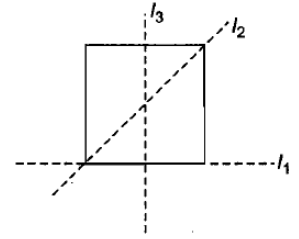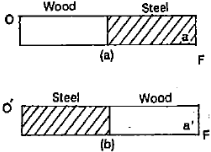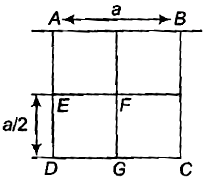If the earth were to suddenly contract to $\frac{1}{n}$th of its present size without any change in its mass, the duration of the new day will be nearly

1.  24/n hours

2.  24 n hours

3.  24/${\mathrm{n}}^{2}$ hours

4.   24 ${\mathrm{n}}^{2}$ hours

Concept Questions :-

Angular momentum
High Yielding Test Series + Question Bank - NEET 2020

Difficulty Level:

A constant torque of 31.4 N-m is exerted on a pivoted wheel. If angular acceleration of wheel is , then the moment of inertia of the wheel is

(1) 2.5 $kg-{m}^{2}$

(2)

(3)

(4)

Concept Questions :-

Torque
High Yielding Test Series + Question Bank - NEET 2020

Difficulty Level:

The moment of inertia of a flywheel having kinetic energy 360 J and angular speed of 20 rad/s is

1.  18 kg${\mathrm{m}}^{2}$

2.   1.8 kg${\mathrm{m}}^{2}$

3.   2.5 kg${\mathrm{m}}^{2}$

4.   9 kg${\mathrm{m}}^{2}$

Concept Questions :-

Angular momentum
High Yielding Test Series + Question Bank - NEET 2020

Difficulty Level:

A sqaure lamina is as shown in figure. The moment of inertia of the frame about the three axes shown in figure are  respectively. Select the correct alternative.(1) ${I}_{2}={I}_{3}>{I}_{1}$

(2) ${I}_{1}>{I}_{2}>{I}_{3}$

(3) ${I}_{2}={I}_{3}<{I}_{1}$

(4) ${I}_{1}<{I}_{2}<{I}_{3}$

Concept Questions :-

Moment of inertia
High Yielding Test Series + Question Bank - NEET 2020

Difficulty Level:

Three interacting particles of masses 1.0 kg, 2.0 kg and 4.0 kg have each a velocity of 1 $m{s}^{-1}$ magnitude along the positive direction of x, y and z-axis respectively. Due to forces of interaction third particle stops and the velocity of second particle becomes , the velocity of the first particle is

(1) $4\stackrel{^}{i}+\stackrel{^}{j}+\stackrel{^}{k}$

(2) $\stackrel{^}{i}-2\stackrel{^}{j}+2\stackrel{^}{k}$

(3) $2\stackrel{^}{i}+3\stackrel{^}{j}-\stackrel{^}{k}$

(4) $-\stackrel{^}{i}+3\stackrel{^}{j}+\stackrel{^}{k}$

Concept Questions :-

Linear momentum
High Yielding Test Series + Question Bank - NEET 2020

Difficulty Level:

A particle performs uniform circular motion with an angular momentum L. If the frequency of the particle motion is double, the angular momentum becomes

(1) 2L

(2) 4L

(3) $\frac{L}{2}$

(4) $\frac{L}{4}$

Concept Questions :-

Angular momentum
High Yielding Test Series + Question Bank - NEET 2020

Difficulty Level:

In figure (a), a meter stick, half of which is wood and the other half steel is pivoted at the wooden end at O and a force F is applied to the steel end a. In figure (b) the stick is pivoted at the steel eid at O' and the same force F is applied at the wooden end at a' The angular acceleration1.  in (a) is greater than in (b)

2.  in (b) is greater than in (a)

3.  equal both in (a) and (b)

4.  none of the above.

Concept Questions :-

Torque
High Yielding Test Series + Question Bank - NEET 2020

Difficulty Level:

A 20 kg solid disc rolls on a horizontal surface at the rate of 4 $m{s}^{-1}$. Its total kinetic energy is

1.  60 J

2.  120 J

3.  240 J

4.  80 J

Concept Questions :-

Rolling motion
High Yielding Test Series + Question Bank - NEET 2020

Difficulty Level:

A sphere rolls down on an inclined plane of inclination $\theta$. What is the acceleration as the sphere reaches the bottom?

1.

2.

3.

4.

Concept Questions :-

Rolling motion
High Yielding Test Series + Question Bank - NEET 2020

Difficulty Level:

From a uniform square plate of side a and mass m, a square portion DEFG of side $\frac{\mathrm{a}}{2}$ is removed. Then, the moment of inertia of the remaining portion about the axis AB is1.

2.

3.

4.

Concept Questions :-

Moment of inertia